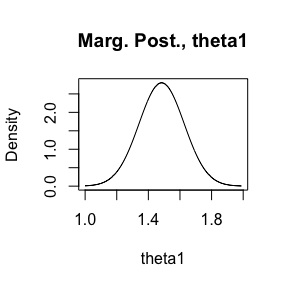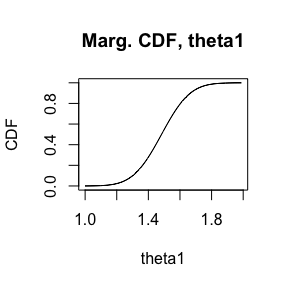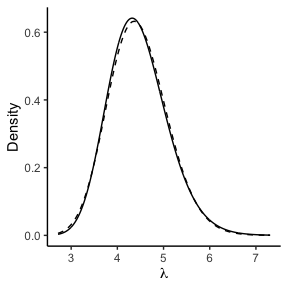# aghq: Adaptive Gauss-Hermite Quadrature for Bayesian inference

library(aghq)
library(dplyr)
#>
#> Attaching package: 'dplyr'
#> The following objects are masked from 'package:stats':
#>
#>     filter, lag
#> The following objects are masked from 'package:base':
#>
#>     intersect, setdiff, setequal, union
library(ggplot2)

This vignette describes the aghq package for Bayesian inference using Adaptive Gauss Hermite Quadrature as described by Bilodeau, Stringer, and Tang (2020). It is taken almost verbatim from Section 2 (Basic Use) of Stringer (2020). It shows how to use the basic functions of the package to implement a very simple example of Bayesian inference using adaptive quadrature. For six challenging and illuminating examples, consult Stringer (2020).

# A simple example

The following conjugate model is used by Bilodeau, Stringer, and Tang (2020): \begin{equation}\begin{aligned} Y_i | \lambda &\overset{ind}{\sim} \text{Poisson}(\lambda), i\in[n], \\ \lambda &\sim \text{Exponential}(1), \lambda > 0, \\ \implies \lambda | Y &\sim \text{Gamma}\left(1 + \sum_{i=1}^{n}y_{i},n+1\right), \\ \pi(\lambda,Y) &= \frac{\lambda^{\sum_{i=1}^{n}y_{i}}e^{-n\lambda}}{\prod_{i=1}^{n}y_{i}!}\times e^{-\lambda}, \\ \pi(Y) &= \frac{\Gamma\left(1 + \sum_{i=1}^{n}y_{i}\right)}{(n+1)^{\left(1 + \sum_{i=1}^{n}y_{i}\right)}\prod_{i=1}^{n}y_{i}!} \end{aligned}\end{equation} We apply a transformation: \begin{equation}\begin{aligned} \eta &= \log\lambda, \\ \implies \log\pi(\eta,Y) &= \eta\sum_{i=1}^{n}y_{i} - (n+1)e^{\eta} - \sum_{i=1}^{n}\log(y_{i}!) + \eta, \\ \end{aligned}\end{equation} which, because the jacobian $$|d\lambda/d\eta|$$ is included, does not change the value of $$\pi(Y)$$.

To approximate $$\pi(Y)$$ and then make Bayesian inferences based on $$\pi(\lambda|Y)$$, first install and load the package:

# Stable version:
# install.packages("aghq")
# Or devel version:
# devtools::install_github("awstringer1/aghq")

We will simulate some data from the model:

set.seed(84343124)
y <- rpois(10,5) # True lambda = 5, n = 10

The main function is the aghq::aghq function. The user supplies a list containing the log-posterior and its first two derivatives:

# Define the log posterior, log(pi(eta,y)) here
logpietay <- function(eta,y) {
sum(y) * eta - (length(y) + 1) * exp(eta) - sum(lgamma(y+1)) + eta
}
# The objective function has to take a single argument, eta, so pass
# in the data:
objfunc <- function(x) logpietay(x,y)
# Simple numerical derivatives suffice here:
objfuncgrad <- function(x) numDeriv::grad(objfunc,x)
objfunchess <- function(x) numDeriv::hessian(objfunc,x)
# Now create the list to pass to aghq()
funlist <- list(
fn = objfunc,
gr = objfuncgrad,
he = objfunchess
)

Now perform the quadrature. The only other required input is the number of quadrature points, $$k$$, and a starting value for the optimization:

# AGHQ with k = 3
# Use eta = 0 as a starting value
thequadrature <- aghq::aghq(ff = funlist,k = 3,startingvalue = 0)

The object thequadrature has class aghq, with summary and plot methods:

summary(thequadrature)
#> AGHQ on a 1 dimensional posterior with  3 quadrature points
#>
#> The posterior mode is: 1.493925
#>
#> The log of the normalizing constant/marginal likelihood is: -23.32123
#>
#> The posterior Hessian at the mode is:
#>      [,1]
#> [1,]   49
#>
#> The covariance matrix used for the quadrature is...
#>            [,1]
#> [1,] 0.02040816
#>
#> ...and its Cholesky is:
#>           [,1]
#> [1,] 0.1428571
#>
#> Here are some moments and quantiles for theta:
#>
#>            mean   median     mode        sd     2.5%    97.5%
#> theta1 1.483742 1.482532 1.493925 0.1424943 1.204135 1.762909
plot(thequadrature)The actual object is a list with elements (see for notation):

• normalized_posterior: the output of the aghq::normalize_posterior function, which is itself a list with elements:

• nodesandweights: a dataframe containing the nodes and weights from the quadrature and the normalized and un-normalized log posterior at the nodes,

• thegrid: a NIGrid object from the mvQuad package, see ?mvQuad::createNIGrid,

• lognormconst: the numeric value of the approximation of the log of the normalizing constant $$\pi(Y)$$.

• marginals: a list of the same length as startingvalue of which element j is the result of calling aghq::marginal_posterior with that j. This is a tbl_df/tbl/data.frame containing the normalized marginal posterior for the $$j^{th}$$ element of $$\eta$$. In this one-dimensional example, this is exactly the same information that is present in thequadrature$normalized_logpost$nodesandweights.

• optresults: information and results from the optimization of $$\log\pi^{*}(\eta,Y)$$. This is itself a list with elements:

• ff: the function list passed to aghq,

• mode: the mode of the log-posterior,

• hessian: the negative Hessian of the log-posterior, at the mode, and

• convergence: the convergence code, specific to the optimizer used.

We can observe the structure of the object and compare the results to the truth for this simple example:

# The normalized posterior:
thequadrature$normalized_posterior$nodesandweights
#>     theta1   weights   logpost logpost_normalized
#> 1 1.246489 0.2674745 -23.67784         -0.3566038
#> 2 1.493925 0.2387265 -22.29426          1.0269677
#> 3 1.741361 0.2674745 -23.92603         -0.6047982
# The log normalization constant:
options(digits = 6)
thequadrature$normalized_posterior$lognormconst
#>  -23.3212
# Compare to the truth:
lgamma(1 + sum(y)) - (1 + sum(y)) * log(length(y) + 1) - sum(lgamma(y+1))
#>  -23.3195
# Quite accurate with only n = 10 and k = 3; this example is very simple.
# The mode found by the optimization:
thequadrature$optresults$mode
#>  1.49393
# The true mode:
log((sum(y) + 1)/(length(y) + 1))
#>  1.49393
options(digits = 3)

Of course, in practical problems, the true mode and normalizing constant won’t be known.

The package provides further routines for computing moments, quantiles, and distributions/densities. These are especially useful when working with transformations and we are in the example, since interest here is in the original parameter $$\lambda = e^{\eta}$$. The main functions are:

• compute_pdf_and_cdf: compute the density and cumulative distribution function for a marginal posterior distribution of a variable and (optionally) a smooth monotone transformation of that variable,

• compute_moment: compute the posterior moment of any function,

• compute_quantiles: compute posterior quantiles for a marginal posterior distribution.

We will consider several examples. To compute the approximate marginal posterior for $$\lambda$$, $$\widetilde{\pi}(\lambda|Y)$$, first compute the marginal posterior for $$\eta$$ and then transform: $\begin{equation} \widetilde{\pi}(\lambda|Y) = \widetilde{\pi}(\eta|Y)|\frac{d\eta}{d\lambda}| \end{equation}$

The aghq::compute_pdf_and_cdf function has an option, transformation, which allows the user to specify a parameter transformation that they would like the marginal density of. The user specifies functions fromtheta and totheta which convert from and to the transformed scale (on which quadrature was done), and the function returns the marginal density on both scales, making use of a numerically differentiated jacobian. This is all done as follows:

# Compute the pdf for eta
pdfwithlambda <- compute_pdf_and_cdf(
thequadrature$marginals[], transformation = list( totheta = function(x) log(x), fromtheta = function(x) exp(x) ) ) # Plot along with the true posterior lambdapostplot <- pdfwithlambda %>% ggplot(aes(x = transparam,y = pdf_transparam)) + theme_classic() + geom_line() + stat_function(fun = dgamma, args = list(shape = 1+sum(y),rate = 1 + length(y)), linetype = 'dashed') + labs(x = expression(lambda),y = "Density") lambdapostplotApproximate (—) and true (- - -) posterior for $$\lambda$$ We may compute the posterior mean of $$\lambda = e^{\eta}$$, $$\EE(\lambda|Y)$$, using the compute_moment function. This can also be used to confirm that the posterior is, in fact, properly normalized: options(digits = 6) # Check if the posterior integrates to 1, by computing the "moment" of "1": compute_moment(thequadrature$normalized_posterior,
ff = function(x) 1)
#>  1
# Posterior mean for eta:
compute_moment(thequadrature$normalized_posterior, ff = function(x) x) #>  1.48374 # Posterior mean for lambda = exp(eta) compute_moment(thequadrature$normalized_posterior,
ff = function(x) exp(x))
#>  4.45441
# Compare to the truth:
(sum(y) + 1)/(length(y) + 1)
#>  4.45455
options(digits = 3)

Quantiles are computed using the compute_quantiles function. For example, to get the first and third percentiles along with median and first and third quartiles:

# Get the quantiles for eta:
etaquants <- compute_quantiles(
thequadrature\$marginals[],
q = c(.01,.25,.50,.75,.99)
)
# The quantiles for lambda = exp(eta) are obtained directly:
exp(etaquants)
#>   1%  25%  50%  75%  99%
#> 3.17 4.00 4.40 4.85 6.15
# and compared with the truth:
qgamma(c(.01,.25,.50,.75,.99),shape = 1+sum(y),rate = 1+length(y))
#>  3.11 4.01 4.42 4.87 6.07

The estimation of quantiles, especially extreme quantiles, is much more difficult than that of moments, and this is reflected in the small differences in accuracy observed between the two in this example.

For more details, see Bilodeau, Stringer, and Tang (2020) and Stringer (2020).